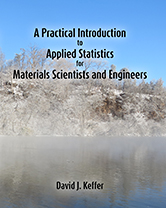A Practical Introduction to Applied Statistics for Materials Scientists and Engineers A Practical Introduction to Applied Statistics for Materials Scientists and Engineers David J. Keffer Professor of Materials Science & Engineering University of Tennessee Knoxville, TennesseeDescription: Materials Scientists and Engineers work in a quantitative field in which the mechanical, thermodynamic, rheological and electronic properties of the materials are described with numbers. Therefore, a basic proficiency in applied statistics is necessary to be able to interpret and communicate the significance and reliability of these numbers. The numbers provide the technical basis upon which are made engineering decisions that impact the successful deployment of the material and ultimately the success of the project, including many elements such as public safety. Understanding the statistical confidence in these numbers is therefore of practical significance. This book provides a practical but fundamental mathematical understanding of applied statistics and the theory of probability that underlies it. This book is targeted at undergraduates. It is used in a course during the third year that covers both applied statistics and numerical methods. Thus, the contents herein are intended to cover half of one semester. The content is divided into six chapters (or modules), covering (1) probability, (2) random variables, (3) expectations, (4) discrete distributions, (5) continuous distributions and (6) sampling and estimation. Other important statistical procedures, such as regression, are covered in the second half of the course, numerical methods. The philosophy espoused in this book is to equip the student with a compact but broadly applicable set of practical problem-solving tools such that the student emerges at the end of the course with the ability to clearly articulate the statistical reliability of the numbers used to describe the materials of interest. Finally, we live in a world where the importance of literacy, the ability to make sense of words, is taken for granted. At the same time, importance of numeracy, the ability to make sense of numbers, is neglected. In fact, poor numeracy skills of the general public (and its elected leaders) are regularly exploited, both intentionally and unintentionally, particularly by those who seek to sway public opinion. An introduction to applied statistics is the first step toward the ability to extract meaning and significance from raw data. In this book, the application of these techniques is toward materials science. However, the broader applicability of such techniques is a natural consequence of one equipped with these skills. This book is currently used to cover the first half of a semester-long course in applied statistics and numerical methods targeted at students in their junior year. The second half of the course, which covers numerical methods, uses a matching textbook, A Practical Introduction to Numerical Methods for Materials Scientists and Engineers. This book assumes a knowledge of differential and integral calculus, with some exposure to multivariate integrals. This book covers: an introduction to probability random variables and probability distributions expectations common discrete probability distribution functions common continuous probability distribution functions sampling, estimation and confidence intervals This book does not cover: regression (included in the Numerical Methods books) analysis of Variance (ANOVA) countless other more advanced statistical topics length: 166 pages paperback size: 8 in x 10 in paperback publication date: September 21, 2015 paperback ISBN-13: 978-1517521936 paperback ISBN: 1517521939 ebook publication date: N.A. ebook ISBN-13: N.A. poison pie publishing house catalog number: PP-031-T